Current Division Rule When current flows through more than one parallel paths, each of the paths shares a definite porion of th...

## Current Division Rule

When current flows through more than one parallel paths, each of the paths shares a definite porion of the total current depending upon the impedance of that path. The definite portion of total current shared by any of the parallel paths can easily be calculated if the impedance of that path and the equivalent impedance of the parallel system are known to us. The rule or formula derived from these known impedances to know the portion of total current through any parallel path is known as current division rule. This rule is very important and widely used in the field of electric engineering in different applications. Actually this rule finds application when we have to find the current passing through each impedance when these are connected in parallel. Let us say, two impedances Z1 and Z2 are connected in parallel as shown below.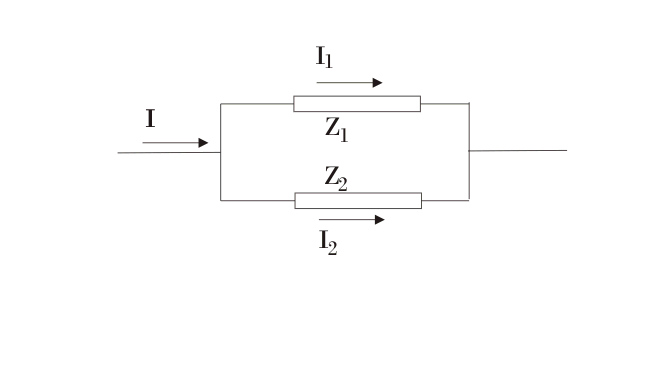A current I passes and is being divided into I1 and I2 at the junction of these two impedances as shown. I1 and I2 pass through Z1 and Z2 respectively. Our aim is to determine I1 and I2 in terms of I, Z1 and Z2.
As Z1 and Z2 are connected in parallel, voltage drop across each will be same. Hence, we can writeAlso applying Kirchoff’s current law at junction, we get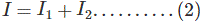We have two equations and can determine I1 and I2.
From (1), we have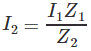Putting this in (2), we get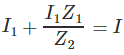or,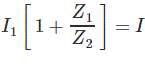or,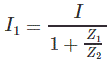or,We have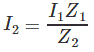Putting value of I1, we get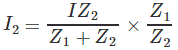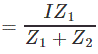Thus, we have determined I1 and I2 in terms of I, Z1 and Z2.
This rule is applied as follows.
Suppose we have to determine I1. We proceed as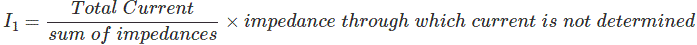Applying above rule, we will get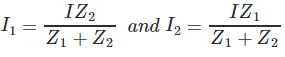Let us apply this rule to some problems.
Let Z1 = 1 + j3, Z2= 3 + j5 and I = 10 Amps.
Applying current divison rule, we will haveWhere I1 = current passing through Z1.
Putting given numerical values, we get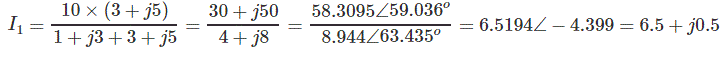Similarly,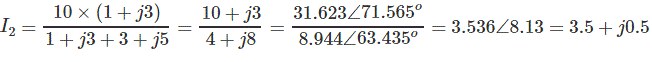The other way to find I2 is as I2 = I – I1 = 10-6.5 + j0.5 = 3.5 + j0.5.
This is how we can apply current division rule.

## Voltage Division Rule

Voltage division rule is applied when we have to find voltage across some impedance. Let us assume that the impedances Z1, Z2, Z3,…..Zn are connected in series and voltage source V is connected across them as shown below.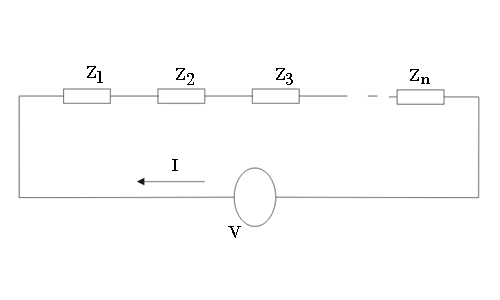Our aim is to find voltage across some impedance, say, Z3. We see that Z1, Z2, Z3 ….Zn are connected in series. Hence, effective impedance Zeff as seen by the voltage is given by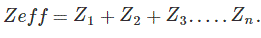Current passing the circuit is given by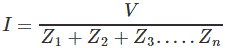This current is passing through all the impedances connected in series. Hence, voltage across Z3 is given by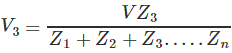Similarly, voltage across Z1 will be given by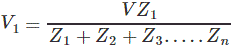In general, we can write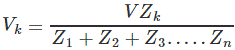Where, k = 1, 2, 3,….n and impedances Z1, Z2, Z3 ,…….Zn should be connected in series.
This is called voltage division rule and frequently used to determine the voltage across some impedance. We can write this rule in words as given below.
Voltage across some impedance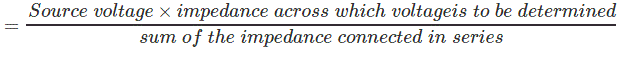Name

BASIC ELECTRICAL,12,BATTERIES,4,CIRCUIT THEORIES,9,CONTROL SYSTEMS,3,DC MOTOR,1,DIGITAL ELECTRONICS,1,DISTRIBUTED GENERATION,2,DISTRIBUTION,6,ELECTRICAL DRIVES,1,ELECTRICAL LAWS,8,ELECTRONICS DEVICES,2,General,7,GENERATION,3,GENERATOR,1,HIGH VOLTAGE,4,ILLUMINATION,1,INDUCTION MOTOR,7,MATERIALS,1,MEASUREMENT,1,MOTOR,1,POWER ELECTRONICS,2,PROJECTS ON INDUCTION MOTOR,1,PROTECTION,1,SMART GRID,3,SWITCHGEAR,4,SYNCHRONOUS MOTOR,1,TRANSFORMER,6,TRANSMISSION,4,
ltr
item
Electrical for Us: Electric Current and Voltage Division Rule
Electric Current and Voltage Division Rule
https://1.bp.blogspot.com/-PhN_3DkuKP0/WEQp-kAQlsI/AAAAAAAAAeE/lHI_7EQiBykRU2TEqz0_RgzNpGnZLC9qACLcB/s320/aid72048-728px-Make-a-Voltage-Divider-Circuit-Step-6.jpg
https://1.bp.blogspot.com/-PhN_3DkuKP0/WEQp-kAQlsI/AAAAAAAAAeE/lHI_7EQiBykRU2TEqz0_RgzNpGnZLC9qACLcB/s72-c/aid72048-728px-Make-a-Voltage-Divider-Circuit-Step-6.jpg
Electrical for Us
https://www.sanjaysah.com.np/2016/12/electric-current-and-voltage-division.html
https://www.sanjaysah.com.np/
http://www.sanjaysah.com.np/
http://www.sanjaysah.com.np/2016/12/electric-current-and-voltage-division.html
true
3851448774078769448
UTF-8